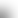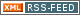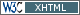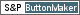www.LinuxHowtos.org howtos, tips&tricks and tutorials for linuxfrom small one page howto to huge articles all in one place

search text in:

Poll
Which screen resolution do you use?

poll results

using iotop to find disk usage hogs

using iotop to find disk usage hogs

words:

887

views:

162276

userrating:

average rating: 1.8 (88 votes) (1=very good 6=terrible)

May 25th. 2007:
Words

486

Views

248050

Workaround and fixes for the current Core Dump Handling vulnerability affected kernels

Workaround and fixes for the current Core Dump Handling vulnerability affected kernels

words:

161

views:

131480

userrating:

average rating: 1.3 (28 votes) (1=very good 6=terrible)

April, 26th. 2006:

You are here: manpages

# bn_internal

Section: OpenSSL (3)
Updated: 2017-05-25

## NAME

bn_mul_words, bn_mul_add_words, bn_sqr_words, bn_div_words, bn_add_words, bn_sub_words, bn_mul_comba4, bn_mul_comba8, bn_sqr_comba4, bn_sqr_comba8, bn_cmp_words, bn_mul_normal, bn_mul_low_normal, bn_mul_recursive, bn_mul_part_recursive, bn_mul_low_recursive, bn_mul_high, bn_sqr_normal, bn_sqr_recursive, bn_expand, bn_wexpand, bn_expand2, bn_fix_top, bn_check_top, bn_print, bn_dump, bn_set_max, bn_set_high, bn_set_low - BIGNUM library internal functions

## SYNOPSIS

``` #include <openssl/bn.h>

BN_ULONG bn_mul_words(BN_ULONG *rp, BN_ULONG *ap, int num, BN_ULONG w);
BN_ULONG bn_mul_add_words(BN_ULONG *rp, BN_ULONG *ap, int num,
BN_ULONG w);
void     bn_sqr_words(BN_ULONG *rp, BN_ULONG *ap, int num);
BN_ULONG bn_div_words(BN_ULONG h, BN_ULONG l, BN_ULONG d);
BN_ULONG bn_add_words(BN_ULONG *rp, BN_ULONG *ap, BN_ULONG *bp,
int num);
BN_ULONG bn_sub_words(BN_ULONG *rp, BN_ULONG *ap, BN_ULONG *bp,
int num);

void bn_mul_comba4(BN_ULONG *r, BN_ULONG *a, BN_ULONG *b);
void bn_mul_comba8(BN_ULONG *r, BN_ULONG *a, BN_ULONG *b);
void bn_sqr_comba4(BN_ULONG *r, BN_ULONG *a);
void bn_sqr_comba8(BN_ULONG *r, BN_ULONG *a);

int bn_cmp_words(BN_ULONG *a, BN_ULONG *b, int n);

void bn_mul_normal(BN_ULONG *r, BN_ULONG *a, int na, BN_ULONG *b,
int nb);
void bn_mul_low_normal(BN_ULONG *r, BN_ULONG *a, BN_ULONG *b, int n);
void bn_mul_recursive(BN_ULONG *r, BN_ULONG *a, BN_ULONG *b, int n2,
int dna,int dnb,BN_ULONG *tmp);
void bn_mul_part_recursive(BN_ULONG *r, BN_ULONG *a, BN_ULONG *b,
int n, int tna,int tnb, BN_ULONG *tmp);
void bn_mul_low_recursive(BN_ULONG *r, BN_ULONG *a, BN_ULONG *b,
int n2, BN_ULONG *tmp);
void bn_mul_high(BN_ULONG *r, BN_ULONG *a, BN_ULONG *b, BN_ULONG *l,
int n2, BN_ULONG *tmp);

void bn_sqr_normal(BN_ULONG *r, BN_ULONG *a, int n, BN_ULONG *tmp);
void bn_sqr_recursive(BN_ULONG *r, BN_ULONG *a, int n2, BN_ULONG *tmp);

void mul(BN_ULONG r, BN_ULONG a, BN_ULONG w, BN_ULONG c);
void mul_add(BN_ULONG r, BN_ULONG a, BN_ULONG w, BN_ULONG c);
void sqr(BN_ULONG r0, BN_ULONG r1, BN_ULONG a);

BIGNUM *bn_expand(BIGNUM *a, int bits);
BIGNUM *bn_wexpand(BIGNUM *a, int n);
BIGNUM *bn_expand2(BIGNUM *a, int n);
void bn_fix_top(BIGNUM *a);

void bn_check_top(BIGNUM *a);
void bn_print(BIGNUM *a);
void bn_dump(BN_ULONG *d, int n);
void bn_set_max(BIGNUM *a);
void bn_set_high(BIGNUM *r, BIGNUM *a, int n);
void bn_set_low(BIGNUM *r, BIGNUM *a, int n);

```

## DESCRIPTION

This page documents the internal functions used by the OpenSSL BIGNUM implementation. They are described here to facilitate debugging and extending the library. They are not to be used by applications.

### The BIGNUM structure

``` typedef struct bignum_st BIGNUM;

struct bignum_st
{
BN_ULONG *d;    /* Pointer to an array of 'BN_BITS2' bit chunks. */
int top;        /* Index of last used d +1. */
/* The next are internal book keeping for bn_expand. */
int dmax;       /* Size of the d array. */
int neg;        /* one if the number is negative */
int flags;
};

```

The integer value is stored in d, a malloc()ed array of words (BN_ULONG), least significant word first. A BN_ULONG can be either 16, 32 or 64 bits in size, depending on the 'number of bits' (BITS2) specified in "openssl/bn.h".

dmax is the size of the d array that has been allocated. top is the number of words being used, so for a value of 4, bn.d=4 and bn.top=1. neg is 1 if the number is negative. When a BIGNUM is 0, the d field can be NULL and top == 0.

flags is a bit field of flags which are defined in "openssl/bn.h". The flags begin with BN_FLG_. The macros BN_set_flags(b,n) and BN_get_flags(b,n) exist to enable or fetch flag(s) n from BIGNUM structure b.

Various routines in this library require the use of temporary BIGNUM variables during their execution. Since dynamic memory allocation to create BIGNUMs is rather expensive when used in conjunction with repeated subroutine calls, the BN_CTX structure is used. This structure contains BN_CTX_NUM BIGNUMs, see BN_CTX_start(3).

### Low-level arithmetic operations

These functions are implemented in C and for several platforms in assembly language:

bn_mul_words(rp, ap, num, w) operates on the num word arrays rp and ap. It computes ap * w, places the result in rp, and returns the high word (carry).

bn_mul_add_words(rp, ap, num, w) operates on the num word arrays rp and ap. It computes ap * w + rp, places the result in rp, and returns the high word (carry).

bn_sqr_words(rp, ap, n) operates on the num word array ap and the 2*num word array ap. It computes ap * ap word-wise, and places the low and high bytes of the result in rp.

bn_div_words(h, l, d) divides the two word number (h,l) by d and returns the result.

bn_add_words(rp, ap, bp, num) operates on the num word arrays ap, bp and rp. It computes ap + bp, places the result in rp, and returns the high word (carry).

bn_sub_words(rp, ap, bp, num) operates on the num word arrays ap, bp and rp. It computes ap - bp, places the result in rp, and returns the carry (1 if bp > ap, 0 otherwise).

bn_mul_comba4(r, a, b) operates on the 4 word arrays a and b and the 8 word array r. It computes a*b and places the result in r.

bn_mul_comba8(r, a, b) operates on the 8 word arrays a and b and the 16 word array r. It computes a*b and places the result in r.

bn_sqr_comba4(r, a, b) operates on the 4 word arrays a and b and the 8 word array r.

bn_sqr_comba8(r, a, b) operates on the 8 word arrays a and b and the 16 word array r.

The following functions are implemented in C:

bn_cmp_words(a, b, n) operates on the n word arrays a and b. It returns 1, 0 and -1 if a is greater than, equal and less than b.

bn_mul_normal(r, a, na, b, nb) operates on the na word array a, the nb word array b and the na+nb word array r. It computes a*b and places the result in r.

bn_mul_low_normal(r, a, b, n) operates on the n word arrays r, a and b. It computes the n low words of a*b and places the result in r.

bn_mul_recursive(r, a, b, n2, dna, dnb, t) operates on the word arrays a and b of length n2+dna and n2+dnb (dna and dnb are currently allowed to be 0 or negative) and the 2*n2 word arrays r and t. n2 must be a power of 2. It computes a*b and places the result in r.

bn_mul_part_recursive(r, a, b, n, tna, tnb, tmp) operates on the word arrays a and b of length n+tna and n+tnb and the 4*n word arrays r and tmp.

bn_mul_low_recursive(r, a, b, n2, tmp) operates on the n2 word arrays r and tmp and the n2/2 word arrays a and b.

bn_mul_high(r, a, b, l, n2, tmp) operates on the n2 word arrays r, a, b and l (?) and the 3*n2 word array tmp.

BN_mul() calls bn_mul_normal(), or an optimized implementation if the factors have the same size: bn_mul_comba8() is used if they are 8 words long, bn_mul_recursive() if they are larger than BN_MULL_SIZE_NORMAL and the size is an exact multiple of the word size, and bn_mul_part_recursive() for others that are larger than BN_MULL_SIZE_NORMAL.

bn_sqr_normal(r, a, n, tmp) operates on the n word array a and the 2*n word arrays tmp and r.

The implementations use the following macros which, depending on the architecture, may use ``long long'' C operations or inline assembler. They are defined in "bn_lcl.h".

mul(r, a, w, c) computes w*a+c and places the low word of the result in r and the high word in c.

mul_add(r, a, w, c) computes w*a+r+c and places the low word of the result in r and the high word in c.

sqr(r0, r1, a) computes a*a and places the low word of the result in r0 and the high word in r1.

### Size changes

bn_expand() ensures that b has enough space for a bits bit number. bn_wexpand() ensures that b has enough space for an n word number. If the number has to be expanded, both macros call bn_expand2(), which allocates a new d array and copies the data. They return NULL on error, b otherwise.

The bn_fix_top() macro reduces a->top to point to the most significant non-zero word plus one when a has shrunk.

### Debugging

bn_check_top() verifies that "((a)->top >= 0 && (a)->top <= (a)->dmax)". A violation will cause the program to abort.

bn_print() prints a to stderr. bn_dump() prints n words at d (in reverse order, i.e. most significant word first) to stderr.

bn_set_max() makes a a static number with a dmax of its current size. This is used by bn_set_low() and bn_set_high() to make r a read-only BIGNUM that contains the n low or high words of a.

If BN_DEBUG is not defined, bn_check_top(), bn_print(), bn_dump() and bn_set_max() are defined as empty macros.

bn(3)

## Index

NAME
SYNOPSIS
DESCRIPTION
The BIGNUM structure
Low-level arithmetic operations
Size changes
Debugging||- Powered by- Running on-
Copyright 2004-2020 Sascha Nitsch Unternehmensberatung GmbH::- Level Triple-A Conformance to Web Content Accessibility Guidelines 1.0 -
- Copyright and legal notices -
Time to create this page: 8.2 ms